## Excel formula: Convert letter to number

Sometimes, you want to convert a to 1, b to 2, c to 3 and so on in Excel sheet. However, to convert them one by one is a waste of time. In this tutorial, I introduce some Excel formulas to solve this problem.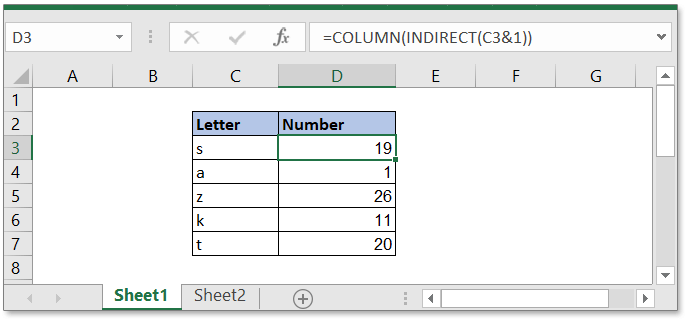### Convert single letter to a number in each cell

To convert single letter to a number in each Excel cell, you can use below formula.

Generic formula:

 COLUMN(INDIRECT(cell_reference&1))

Arguments

 Cell_reference: the cell that contains the letter you want to convert to number.

How this formula work

Take an example to explain the formula. Here you will convert the letter in cell C3 to number. Please use this formula.

 =COLUMN(INDIRECT(C3&1))

Press Enter key.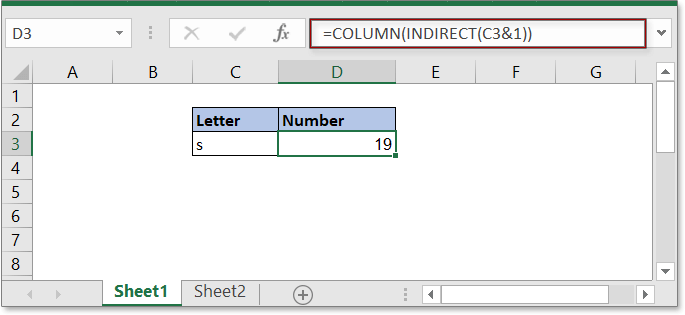Explanation

INDIRECT functiin: this function used to convert a text string to a valid reference. Here INDIRECT(C3&1) you can see it as these:
INDIRECT(C3&1)
=INDIRECT(s&1)
=INDIRECT(s1)

COLUMN function: the COLUMN function returns the number of column of the given reference. Now the formula is COLUMN(s1) which returns 19.

Remarks:

1. This formula returns the number of column, which means that if there are more than one letter in cell, it will return as below example shown.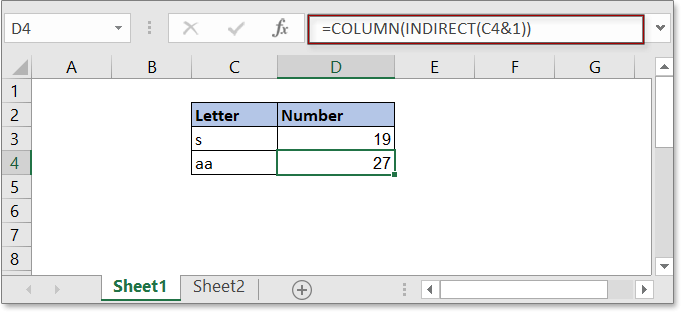2. The formula is case insensitive.

### Convert several letters to a string of numbers in each cell

If you want to convert letters in each cell to numbers as below screenshot shown, you need a complex array formula.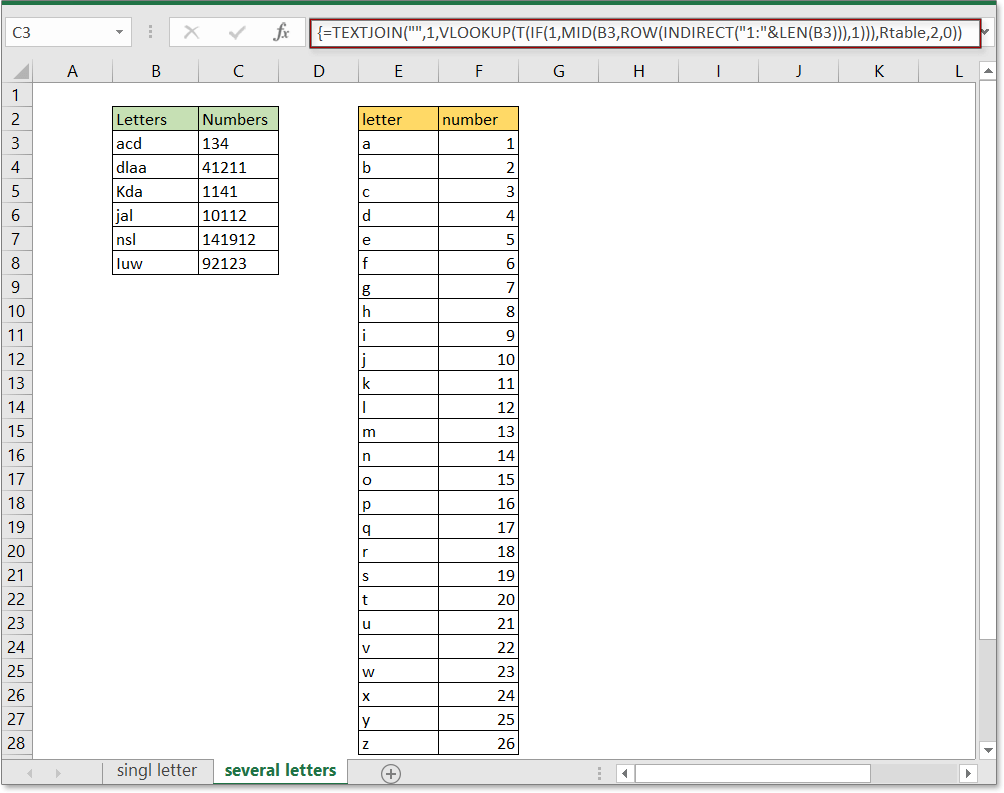Generic formula:

 TEXTJOIN("",1,VLOOKUP(T(IF(1,MID(cell_refer,ROW(INDIRECT("1:"&LEN(cell_refer))),1))),Rtable,2,0))

Arguments

 Cell_refer: the cell that you want to convert the letters to numbers. Rtable:a table with two columns, one lists all letters, one lists the relative numbers.

How this formula work

Supposing you want to convert the letters in range B3:B8 to numbers, please do as these:

Firstly, create a table to list the all letters and their relative numbers.

Then select the table range and go to the Name box (beside the Formula bar) to give it a ranged name, here the range name is Rtable. see screenshot: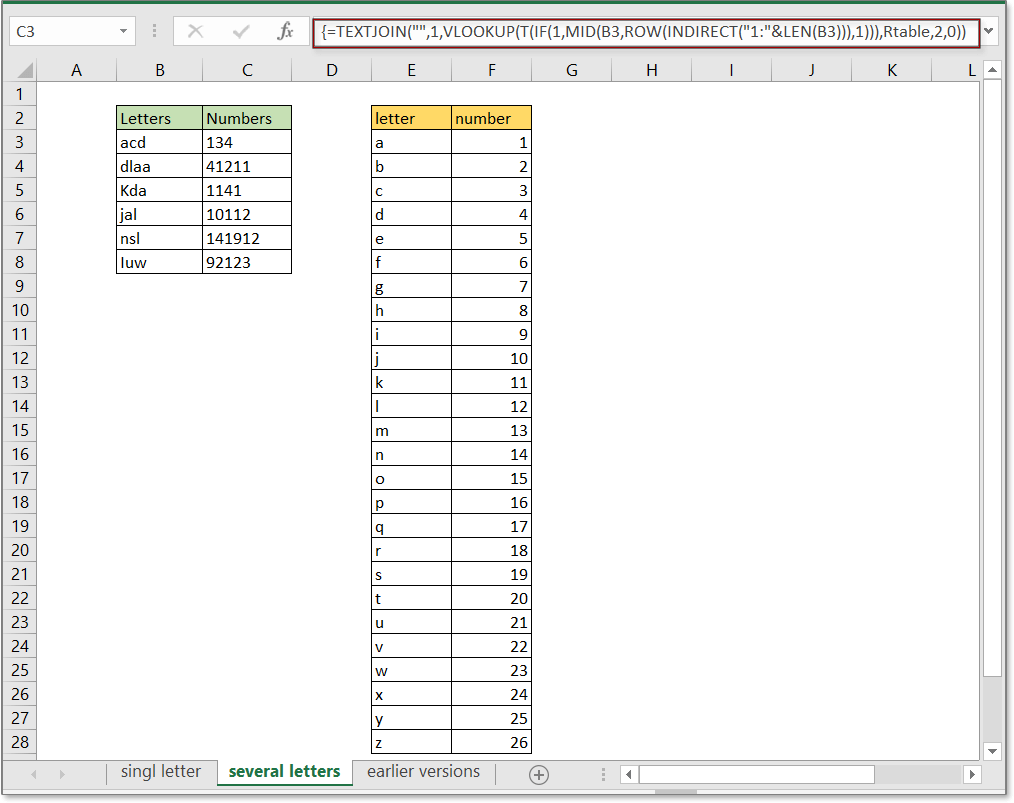Now use this formula:

 =TEXTJOIN("",1,VLOOKUP(T(IF(1,MID(B3,ROW(INDIRECT("1:"&LEN(B3))),1))),Rtable,2,0))

Press Enter key, all first letters of each word in cell A1 is extracted.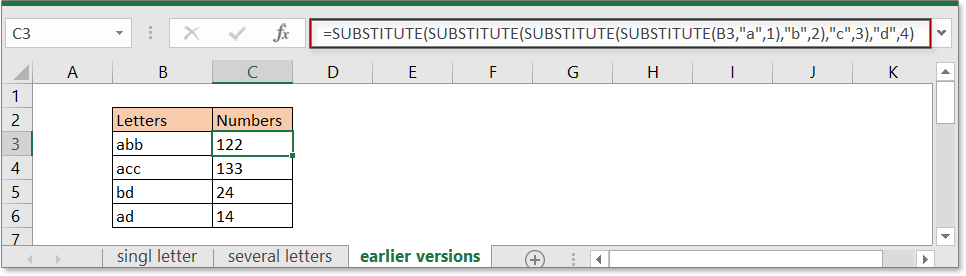Explanation

ROW(INDIRECT("1:"&LEN(B3))): the ROW function returns the number of row, there is the array formula, then it returns {1;2;3}. MID(B3,ROW(INDIRECT("1:"&LEN(B3))),1): the MID function returns the character in the specific position of the given string. This formula can be seen as below
=MID(B3,{1;2;3},1)
=MID(“acd”,{1;2;3},1)
= {"a";"c";"d"}

VLOOKUP(T(IF(1,MID(B3,ROW(INDIRECT("1:"&LEN(B3))),1))),Rtable,2,0): the VLOOKUP function is used to find the matched numbers of "a", "c","d" in the second column of range Rtable. Then it returns {1;3;4}.

TEXTJOIN("",1,VLOOKUP(T(IF(1,MID(B3,ROW(INDIRECT("1:"&LEN(B3))),1))),Rtable,2,0)): the TEXTJOIN function is a new function in Excel 2019 and 365, it is used to concatenated all texts with a delimiter. Here it returns 134.

Remarks:

This formula is case insensitive.

Note:

Supposing a=1,b=2,c=3,d=4, you can use the formula as below:

 =SUBSTITUTE(SUBSTITUTE(SUBSTITUTE(SUBSTITUTE(B3,"a",1),"b",2),"c",3),"d",4)

Press Enter key.This formula is case sensitive.

### Sample File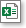Click to download sample file

#### Relative Functions

• INDIRECT function
Convert a text string to a valid reference.
• COLUMN function
Return the number of column which formula appears or the column number of given reference.
• ROW function
Return row number of a reference.
• MID
Return the specific characters from the middle of text string .

### The Best Office Productivity Tools

#### Kutools for Excel - Helps You To Stand Out From Crowd

 Popular Features: Find, Highlight or Identify Duplicates  |  Delete Blank Rows  |  Combine Columns or Cells without Losing Data  |  Round without Formula ... Super VLookup: Multiple Criteria  |  Multiple Value  |  Across Multi-Sheets  |  Fuzzy Lookup... Adv. Drop-down List: Easy Drop Down List  |  Dependent Drop Down List  |  Multi-select Drop Down List... Column Manager: Add a Specific Number of Columns  |  Move Columns  |  Toggle Visibility Status of Hidden Columns  |  Compare Columns to Select Same & Different Cells ... Featured Features: Grid Focus  |  Design View  |  Big Formula Bar  |  Workbook & Sheet Manager | Resource Library (Auto Text)  |  Date Picker  |  Combine Worksheets  |  Encrypt/Decrypt Cells  |  Send Emails by List  |  Super Filter  |  Special Filter (filter bold/italic/strikethrough...) ... Top 15 Toolsets:  12 Text Tools (Add Text, Remove Characters ...)  |  50+ Chart Types (Gantt Chart ...)  |  40+ Practical Formulas (Calculate age based on birthday ...)  |  19 Insertion Tools (Insert QR Code, Insert Picture from Path ...)  |  12 Conversion Tools (Numbers to Words, Currency Conversion ...)  |  7 Merge & Split Tools (Advanced Combine Rows, Split Excel Cells ...)  |  ... and more

Kutools for Excel Boasts Over 300 Features, Ensuring That What You Need is Just A Click Away...#### Office Tab - Enable Tabbed Reading and Editing in Microsoft Office (include Excel)

• One second to switch between dozens of open documents!
• Reduce hundreds of mouse clicks for you every day, say goodbye to mouse hand.
• Increases your productivity by 50% when viewing and editing multiple documents.
• Brings Efficient Tabs to Office (include Excel), Just Like Chrome, Edge and Firefox.No ratings yet. Be the first to rate!
This comment was minimized by the moderator on the site
ฉันไม่เข้าใจ ว่า เราจะกำหนดตัวเลขอย่างไร

COLUMN ฟังก์ชัน: COLUMN ฟังก์ชันส่งคืนจำนวนคอลัมน์ของการอ้างอิงที่ระบุ ตอนนี้สูตรคือ คอลัมน์ (s1) ซึ่งส่งกลับ 19.

เเล้วเราใส่สูตรตรงไหน ที่บอกว่า ข้อความนี้ กำหนด ให้เป็น 19 คะ
This comment was minimized by the moderator on the site
Hello,
I have as a basis this formula: =TEXTVERKETTEN("";1;SVERWEIS(T(IF(1;PART(B3;LINE(INDIRECT("1:"&LENGTH(B3)));1));E3:F78;2;0)) ... I have expanded my table in upper and lower case including special characters. Unfortunately, I do not get the difference verstädnlich integrated into the above formula!?

Helpful would still be the generic formula retroactively. That is, if I enter the numerical order, the table gives me the text.

I would like to use the generic formula for password encryption.